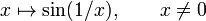# Sine of reciprocal function

For functions involving angles (trigonometric functions, inverse trigonometric functions, etc.) we follow the convention that all angles are measured in radians. Thus, for instance, the angle of$90\,^\circ$ is measured as$\pi/2$.$x \mapsto \sin(1/x), \qquad x \ne 0$